# 1 Python Line for ELMo Word Embeddings and t-SNE plots with John Snow Labs’ NLU

Including Part of Speech, Named Entity Recognition, Emotion, and Sentiment Classification in the same line! With Bonus t-SNE plots and comparison of various ELMo output layers!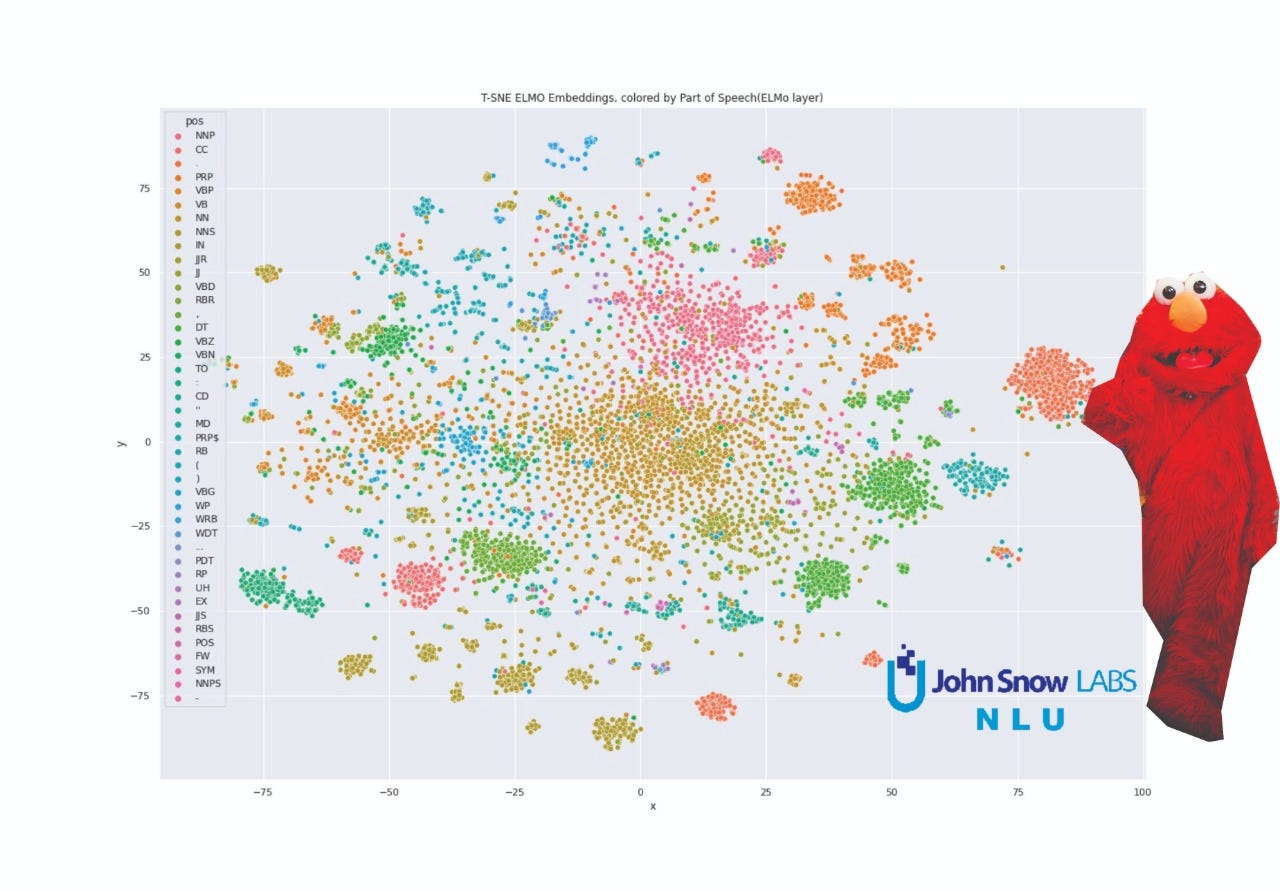T-SNE visualization for ELMo Embeddings. This is one of the plots we will end up at the end of this tutorial!

# 0.1 What is NLU?

John Snow Labs NLU library gives you 350+ NLP models and 100+ Word Embeddings and infinite possibilities to explore your data and gain insights.

In this tutorial, we will cover how to get the powerful ELMo Embeddings with 1 line of NLU code and then how to visualize them with t-SNE. We will compare Comparing Sentiment with Sarcasm and Emotions!

# 0.2 What is t-SNE?

T-SNE is a tool to visualize high-dimensional data. It converts similarities between data points to joint probabilities and tries to minimize the Kullback-Leibler divergence between the joint probabilities of the low-dimensional embedding and the high-dimensional data. t-SNE has a cost function that is not convex, i.e. with different initializations we can get different results.

# 0.3 How does ELMo differ from past approaches?

ELMo, created by AllenNLP broke the state of the art (SOTA) in many NLP tasks upon release. Together with ULMFiT and OpenAi, ELMo brought upon us NLP’s breakthrough imagenet moment. These embedding techniques were a great step forward better results compared to older methods like word2vec or GloVe.

# 0.4 How does it differ from newer models like BERT, XLNET, or ALBERT?

In contrast to BERT, XLNET, and ALBERT which are trained on masking random words in a sentence, ELMo is trained on predicting the next word in a sequence. ELMo is relying on bidirectional LSTM’s under the hood and is not transformer-based, like BERT, XLNET, ALBERT, and USE. In case you wanna try them out, they are all available in Spark NLP as annotators! We will cover them in the upcoming tutorials.

# 1. How to get ELMo embeddings in 1 line?

`nlu.load('elmo').predict(youData)`

That's all you need! Make sure you ran previously

`pip install nlu`

Since adding additional classifiers and getting their predictions is so easy in NLU, we will extend our NLU pipeline with a POS, Emotion, and Sentiment classifier which all achieve results close to the state of the art.

Those extra predictions will also come in handy when plotting our results.

`pipe = nlu.load('pos sentiment elmo emotion').predict(df)`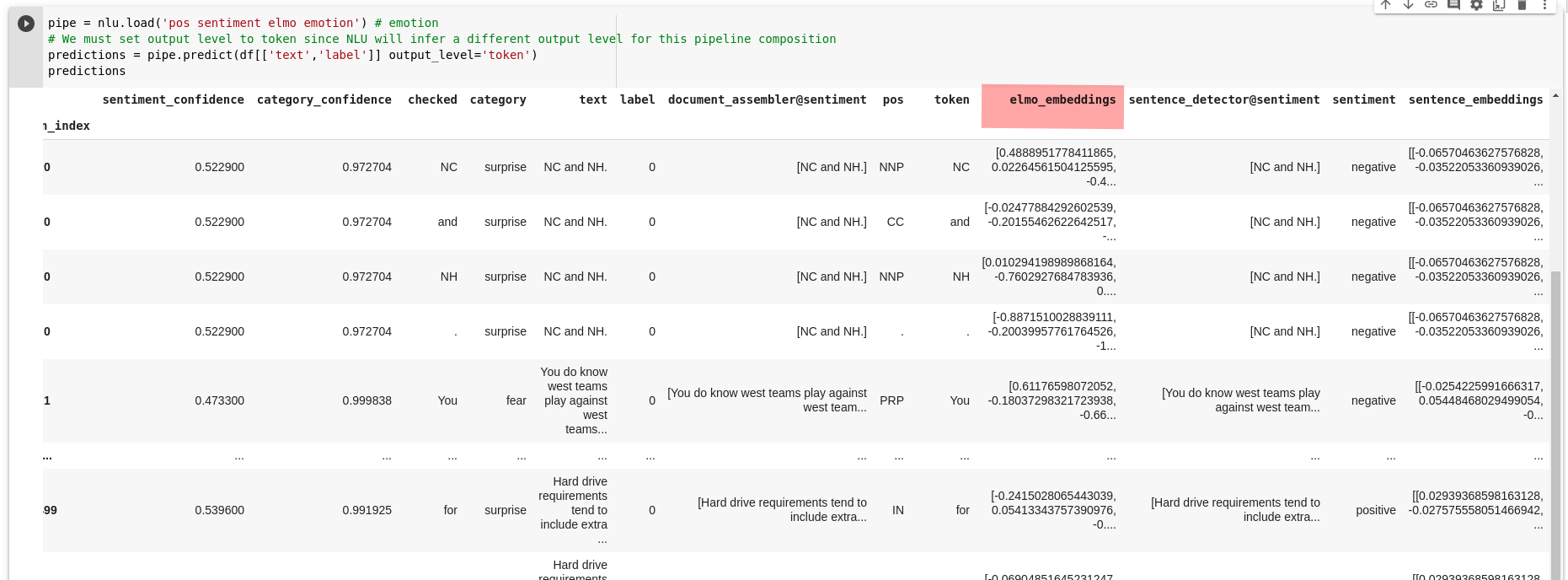We added Part of Speech(POS), Sentiment, and also Emotion classification as bonus into the same line and receive a pandas dataframe rich with features

# 2. Prepare data for T-SNE

We prepare the data for the T-SNE algorithm by collecting them in a matrix for TSNE

`import numpy as npmat = np.matrix([x for x in predictions.elmo_embeddings])`

# 3. Fit T-SNE

Finally, we fit the T-SNE algorithm and get our 2-Dimensional representation of our Bert Word Embeddings

`TSNEmodel = TSNE(n_components=2)low_dim_data = model.fit_transform(mat)print('Lower dim data has shape',low_dim_data.shape)`

# 4. Plot ELMo Word Embeddings, colored by Part of Speech Tag

The following plots show scatter plots for the 2-D representation of the Word Embeddings. Each point represents a word in a sentence and the color represents the POS class that word belongs to.

`tsne_df =  pd.DataFrame(low_dim_data, predictions.pos)tsne_df.columns = ['x','y']ax = sns.scatterplot(data=tsne_df, x='x', y='y', hue=tsne_df.index)ax.set_title('T-SNE ELMO Embeddings, colored by Part of Speech Tag')`

# 5. Plot emotional distribution

Since we added emotion classification, why not plot the distribution of it in 1 line quickly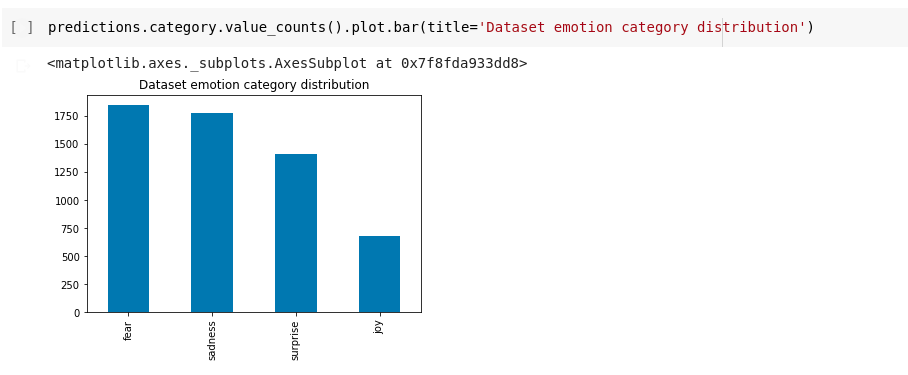We can really quickly plot the emotional distribution of our dataset, mostly negative feelings in our dataset

# 6. Try out Elmo’s different output pooling layers

Elmo has been released with 4 different output layers accessible to us. Each of them encodes tokens and their contextual meaning differently. It can be very interesting to experiment with them and compare their different t-SNE embeddings and how they perform in various NLP downstream tasks.

• word_emb: the character-based word representations with shape [batch_size, max_length, 512]
• lstm_outputs1: the first LSTM hidden state with shape [batch_size, max_length, 1024]
• lstm_outputs2: the second LSTM hidden state with shape [batch_size, max_length, 1024]
• elmo: the weighted sum of the 3 layers, where the weights are trainable. This tensor has shape [batch_size, max_length, 1024]

Refer to the paper for more specific info about the pooling layers.

The following code snippet will print for us every component in our nlu pipeline and also copy pastable code we can use to configure our model

`pipe.print_info()`

Will print:

# Change Elmos output Layer

We can just copy-paste the .setPoolingLayer() line and put ‘elmo’ or any other of the 4 layers as parameters and then predict with the configured pipe.

`pipe['elmo'].setPoolingLayer('elmo')predictions = pipe.predict(df)`

Afterward, we can run again the plotting code, which is quite short if you put it in the code block

`mat = np.matrix([x for x in predictions.elmo_embeddings])low_dim_data = model.fit_transform(mat)tsne_df =  pd.DataFrame(low_dim_data, predictions.pos)tsne_df.columns = ['x','y']ax = sns.scatterplot(data=tsne_df, x='x', y='y', hue=tsne_df.index)ax.set_title('T-SNE ELMO Embeddings, colored by Part of Speech Tag for Elmo Layer')`

I had some fun with this and ran all layers and plotted them with a different hue (Part of Speech, Emotion, Sentiment, Sarcasm) enjoy!

# ElMo t-SNE plots for Part of Speech coloring

In case you are curious about what each of the Part of Speech tags in the plot legend stands for, you can find every NER tag described and with an example in the NLU docs.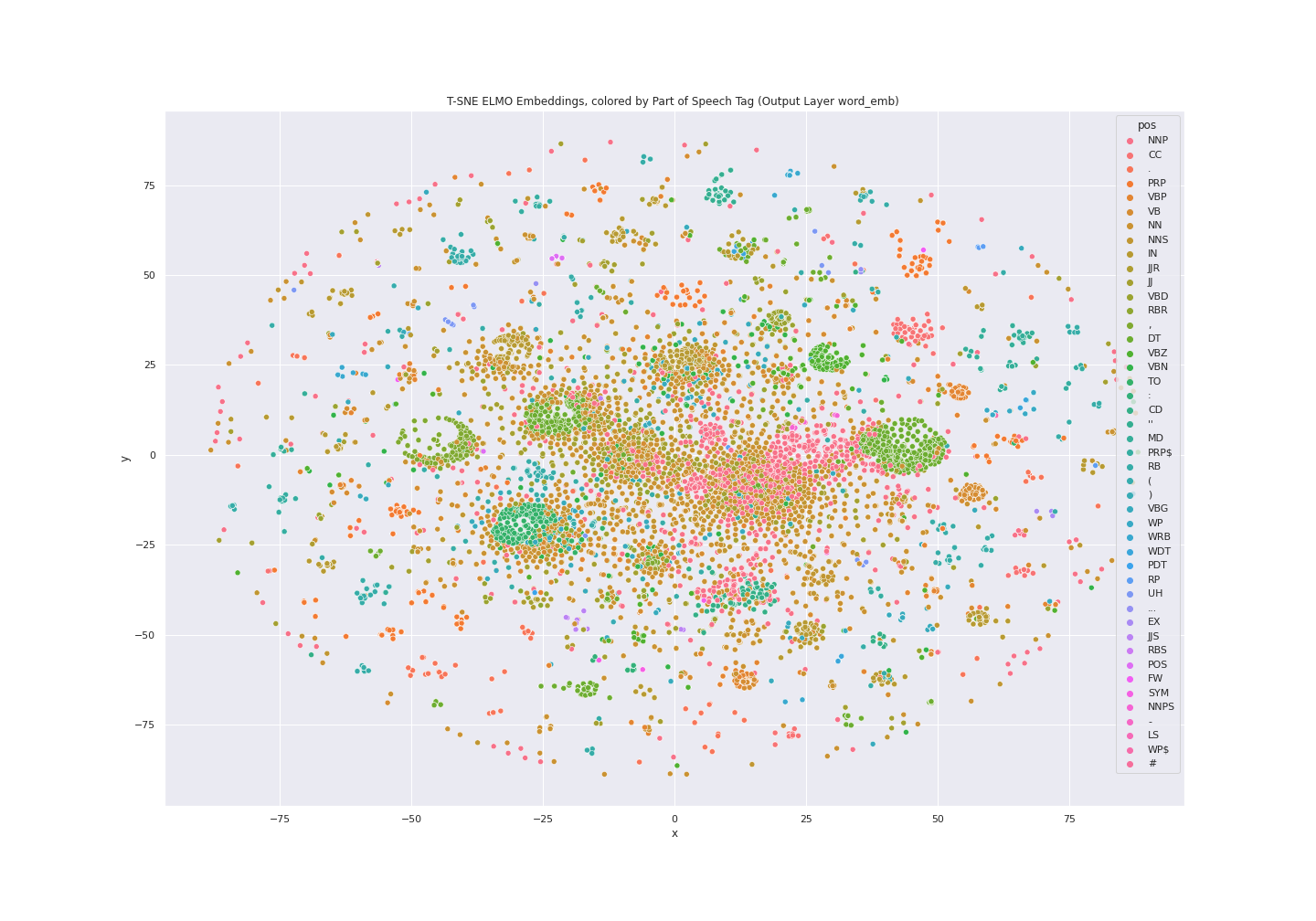t-SNE plot for ELMo output layer Word Embedding colored by Part Of Speech (POS)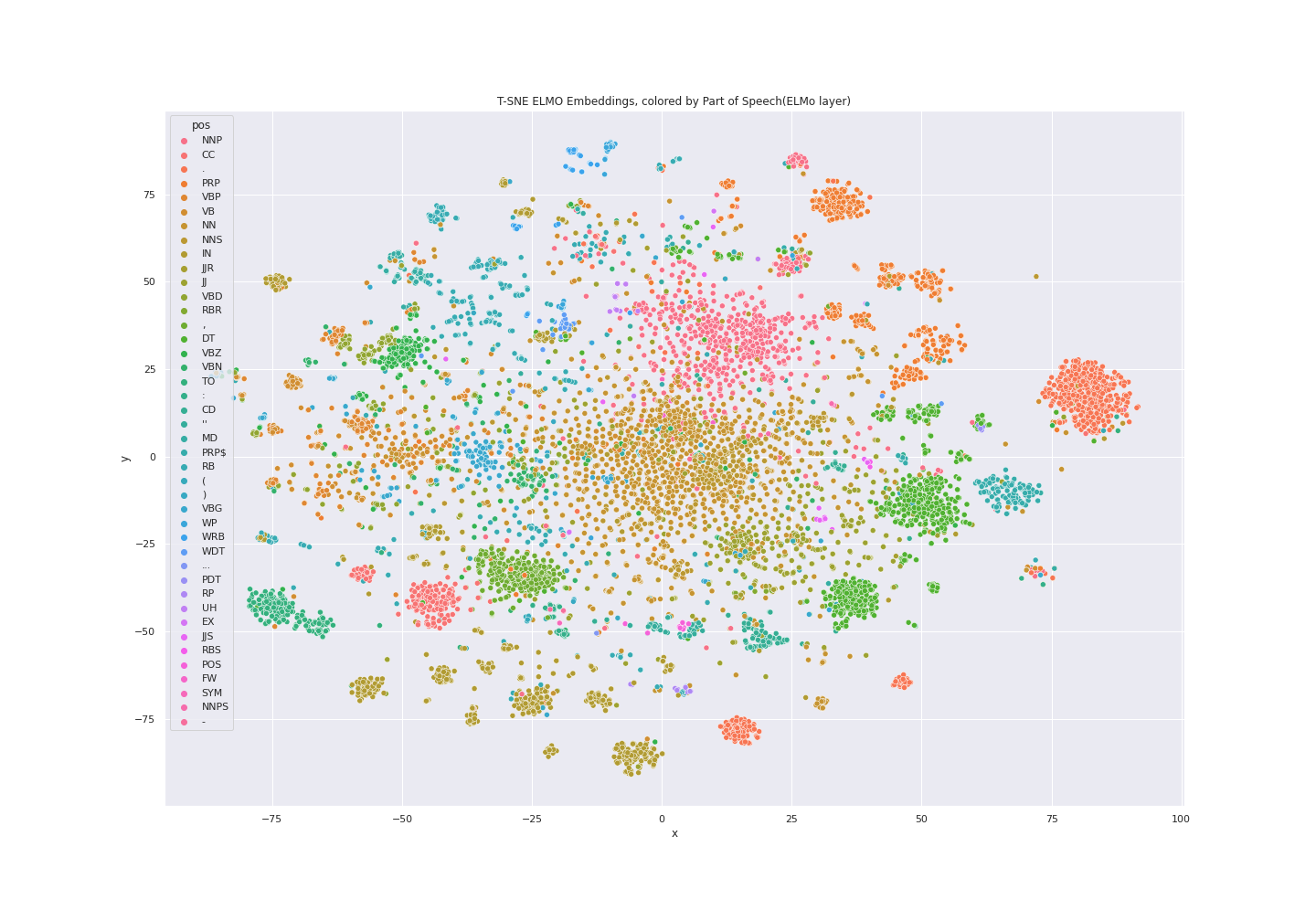t-SNE plot for ELMo output layer elmo colored by Part Of Speech (POS)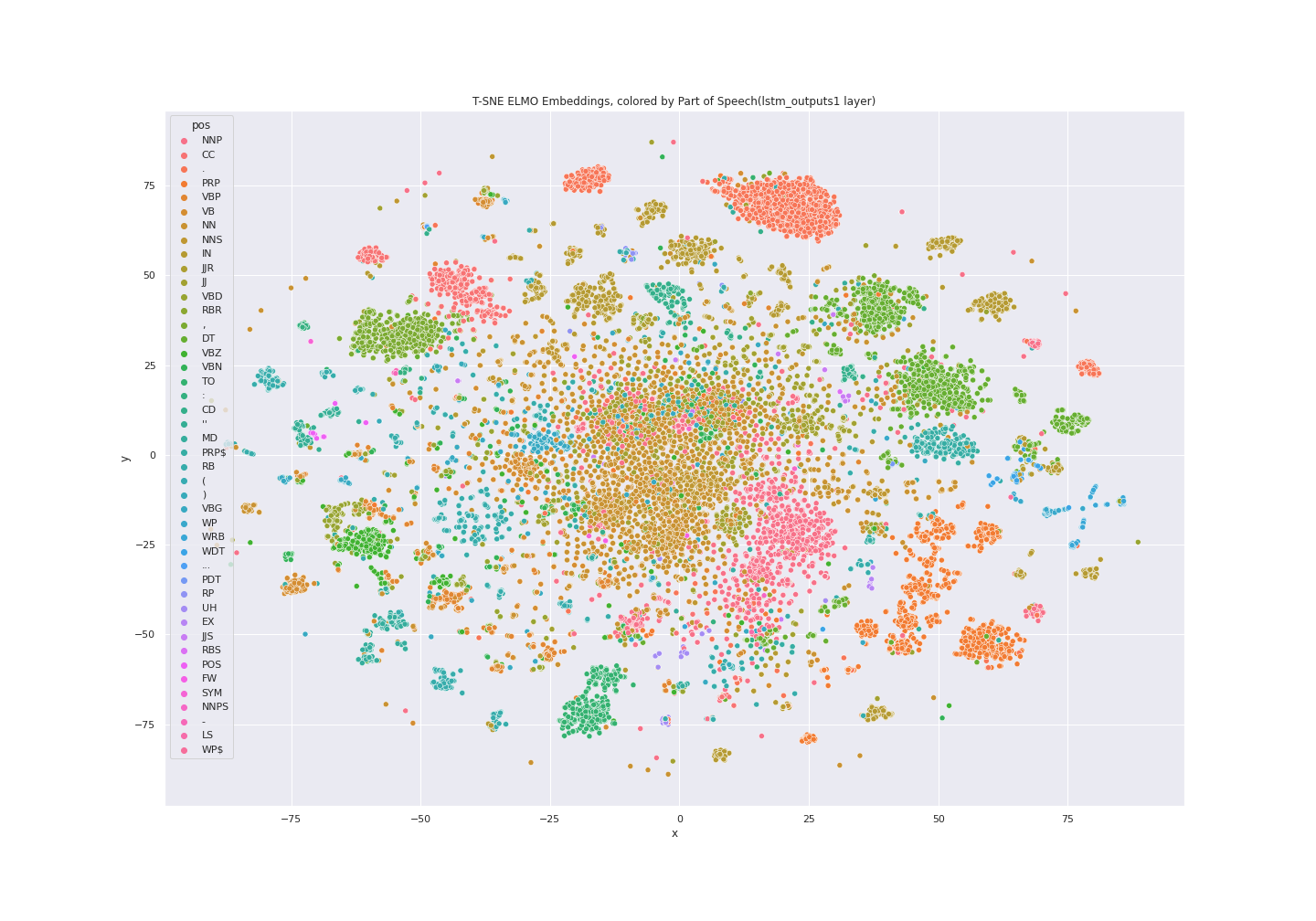t-SNE plot for ELMo output layer lstm1 colored by Part Of Speech (POS)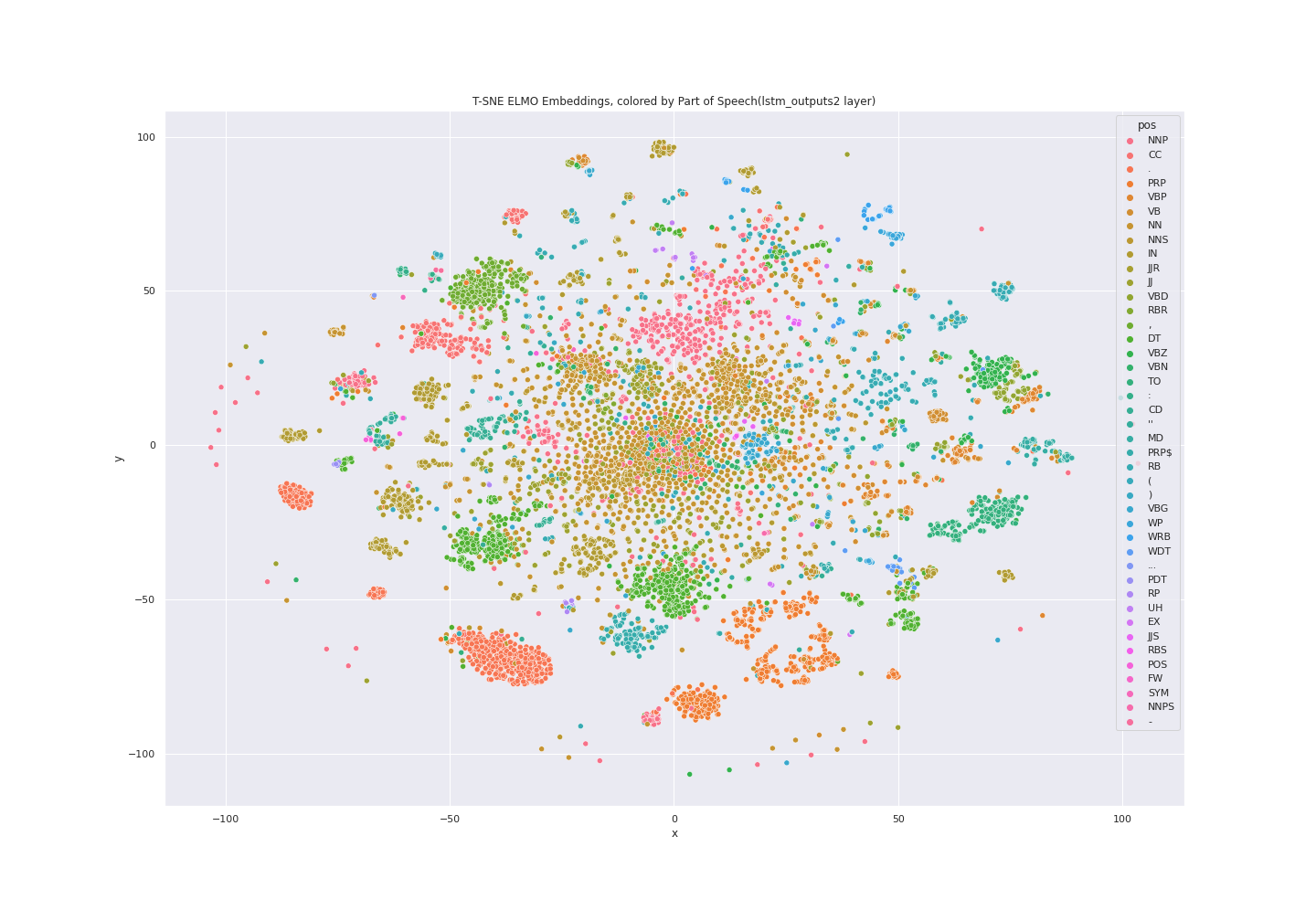t-SNE plot for ELMo output layer lstm2 colored by Part Of Speech (POS)

# All ELMo plots for output layer LSTM2 together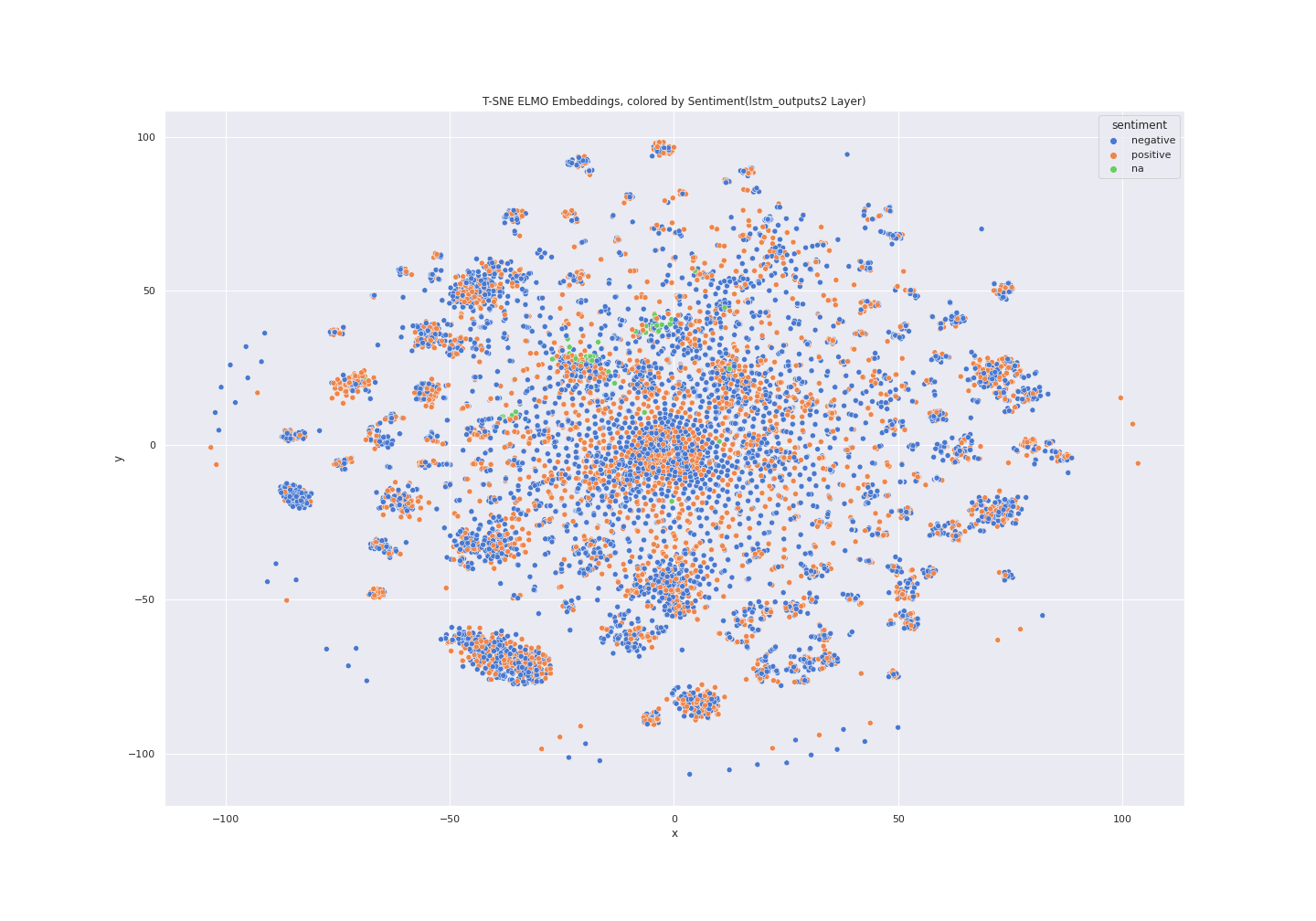Left emotion and right sentiment for LSTM2 output layer of ELMo

# What’s the full code to generate the t-SNE plots?

You really just need 1 line of NLU code and a few sprinkles of plotting and TSNE code showcased in the following code segment

`nlu.load('elmo').predict(youData)model = TSNE(n_components=2)mat = np.matrix([x for x in predictions.elmo_embeddings])low_dim_data = model.fit_transform(mat)tsne_df =  pd.DataFrame(low_dim_data, predictions.pos)tsne_df.columns = ['x','y']ax = sns.scatterplot(data=tsne_df, x='x', y='y', hue=tsne_df.index)ax.set_title('T-SNE ELMO Embeddings, colored by Part of Speech Tag')`

# What if I want to work with terabytes of big data?

We had to limit ourselves to a subsection of the dataset because our RAM is sadly limited with just one machine.
With Spark NLP you could take exactly the same models and run them in a scalable fashion inside of a Spark cluster on terabytes of data because NLU is using Spark NLP under the hood to generate its predictions!